# 一类含五次非线性恢复力的Duffing系统共振与分岔特性分析*

(南昌工学院 理学院， 南昌330108)

## 1 系统响应求解及共振特性分析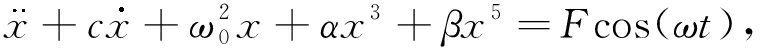(1)

x=x0(T0,T1)+εx1(T0,T1)

(2)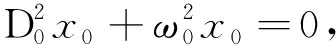(3)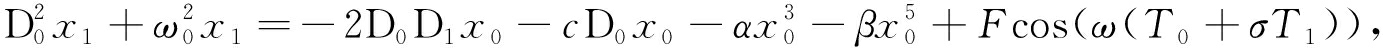(4)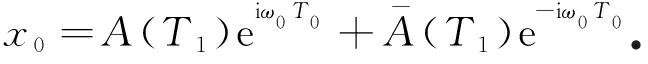(5)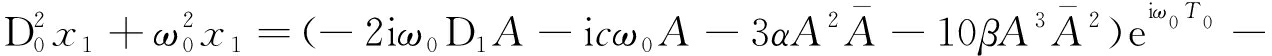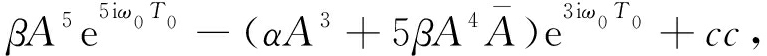(6)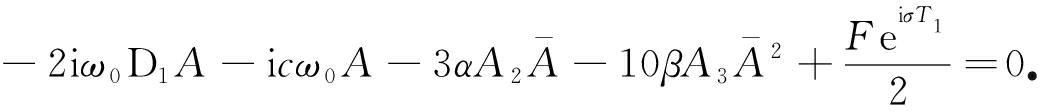(7)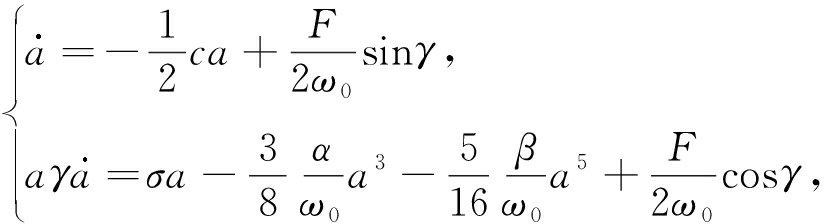(8)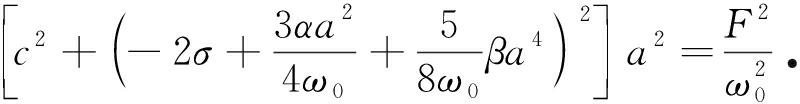(9)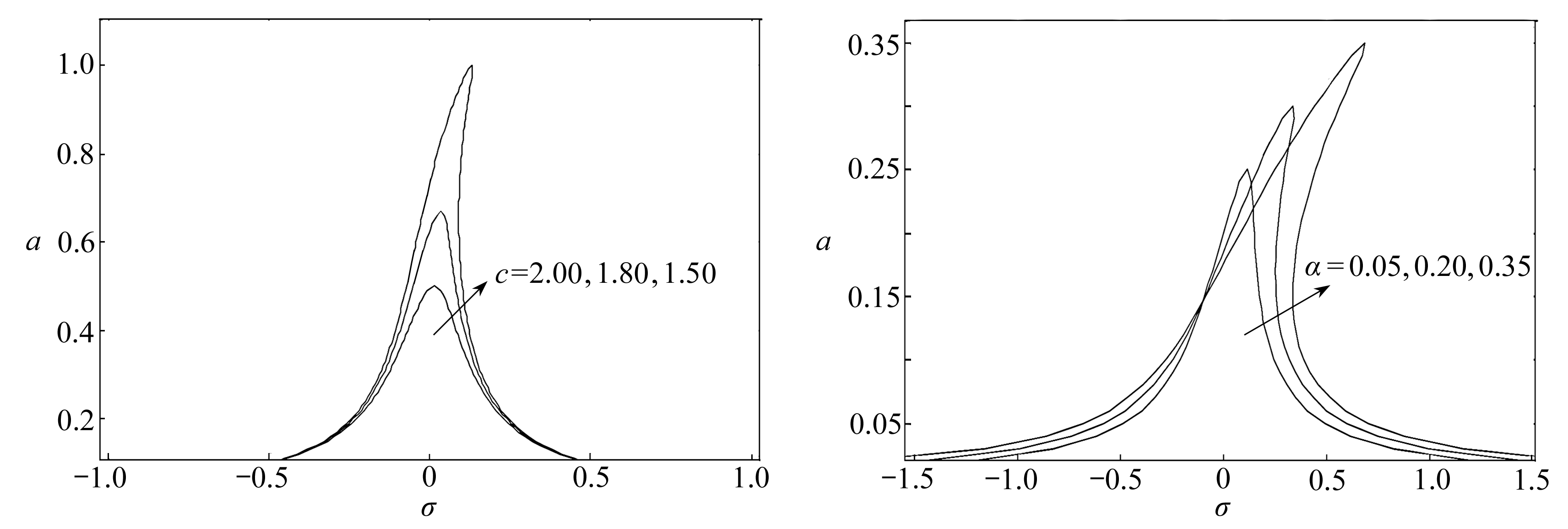Fig. 1 The influence of linear damping Fig. 2 The influence of cubic nonlinear
coefficient c on the system coefficient α on the system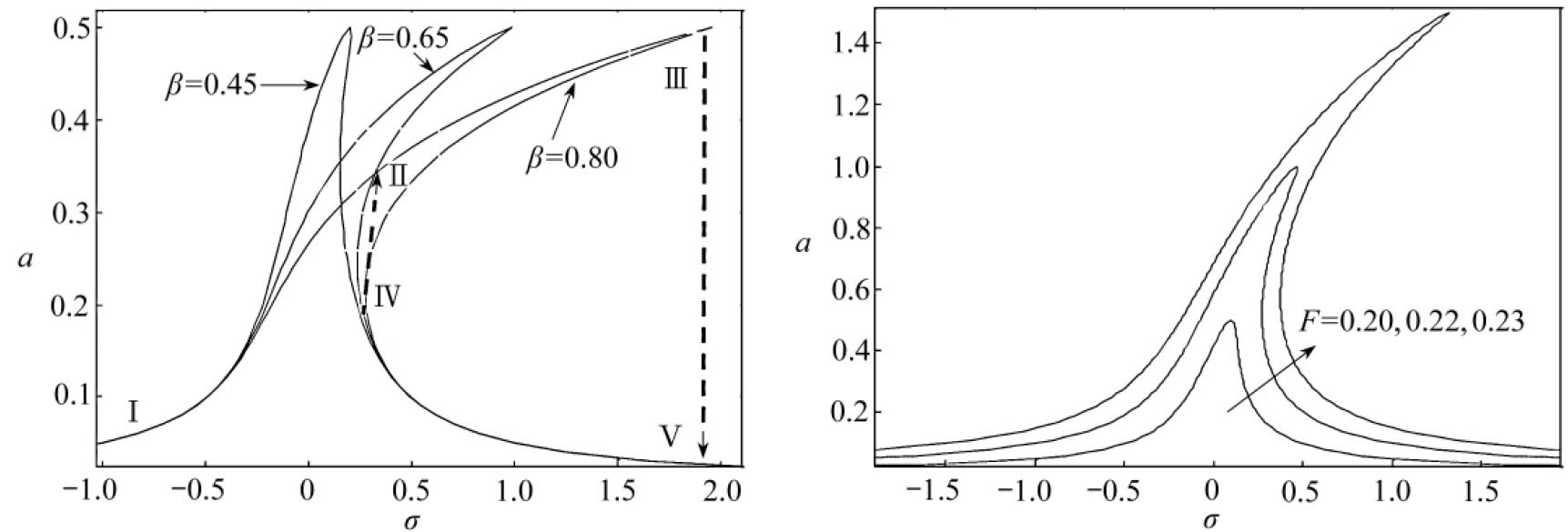Fig. 3 The influence of quintic nonlinear coefficient Fig. 4 The influence of excitation amplitude
β on the system F on the system

## 2 静态分岔特性分析

l5a10+l4a8+l3a6+l2a4+l1a2+l0=0，

(10)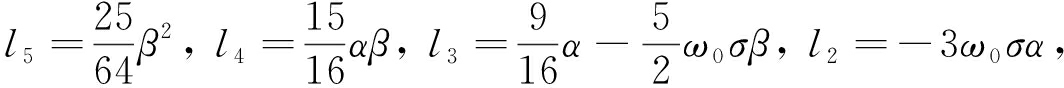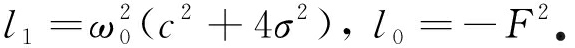b=a2，则式(10)可化为

l5b5+l4b4+l3b3+l2b2+l1b+l0=0

(11)

μ5+λ+δ1μ+δ2μ2+δ3μ3=0，

(12)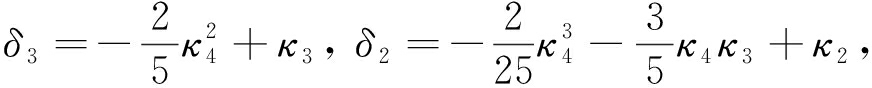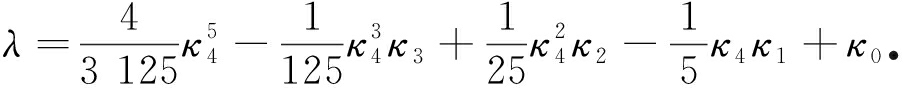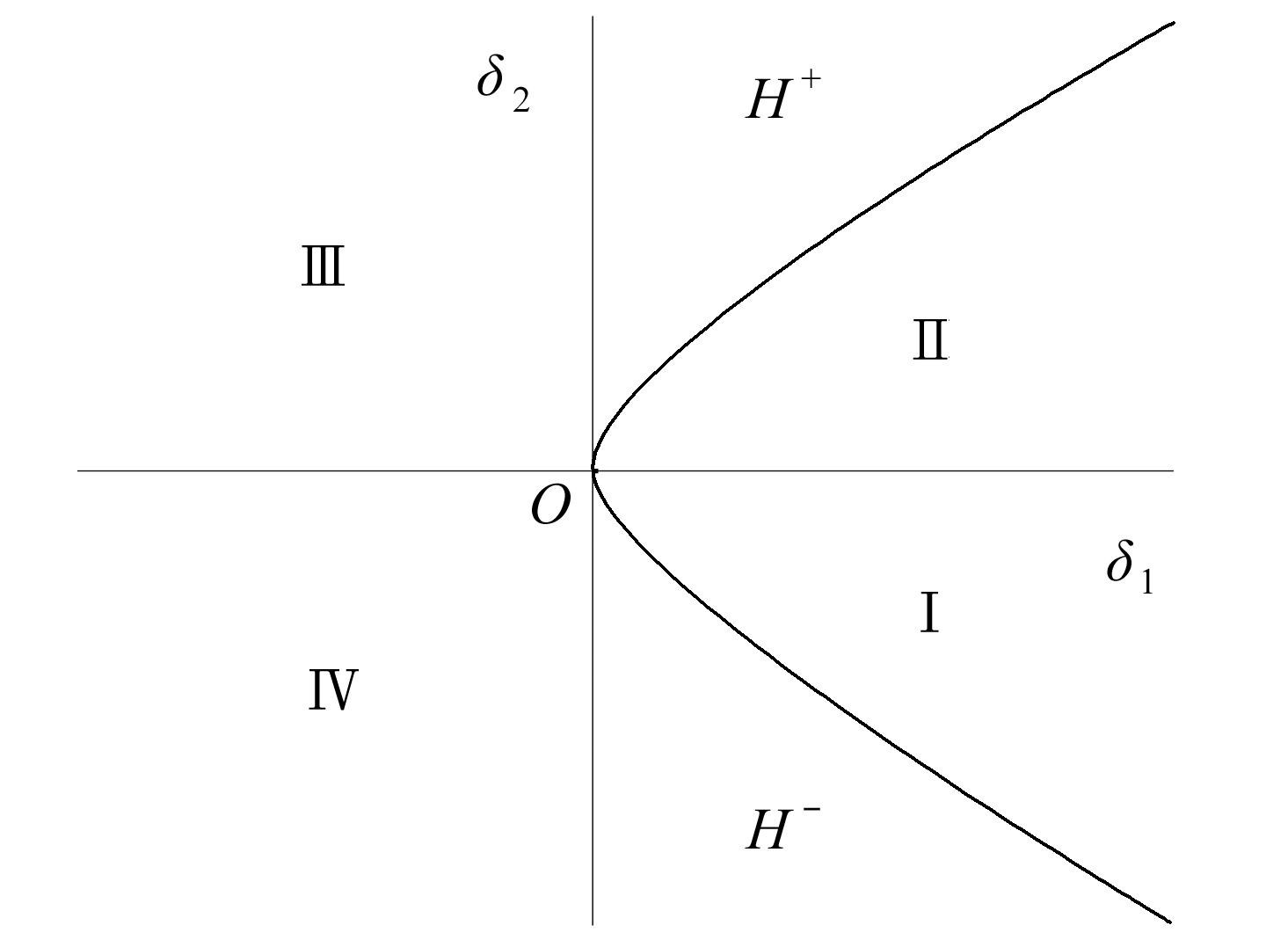Fig. 5 The transition set for δ3=0

δ3=0

δ3=0时，可得系统分岔点集B=∅(∅为空集)，滞后点集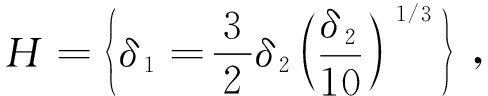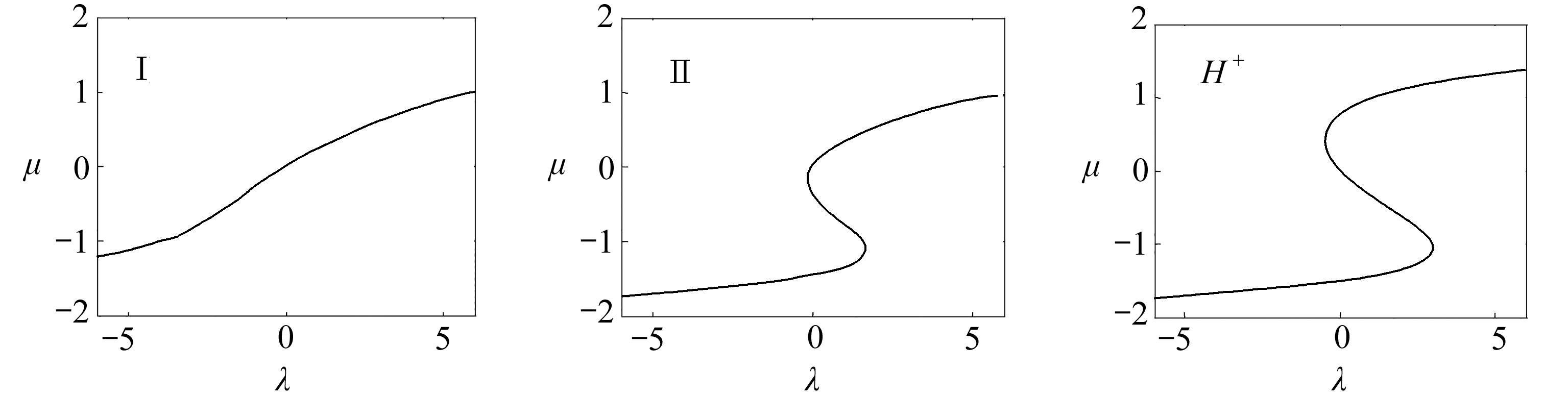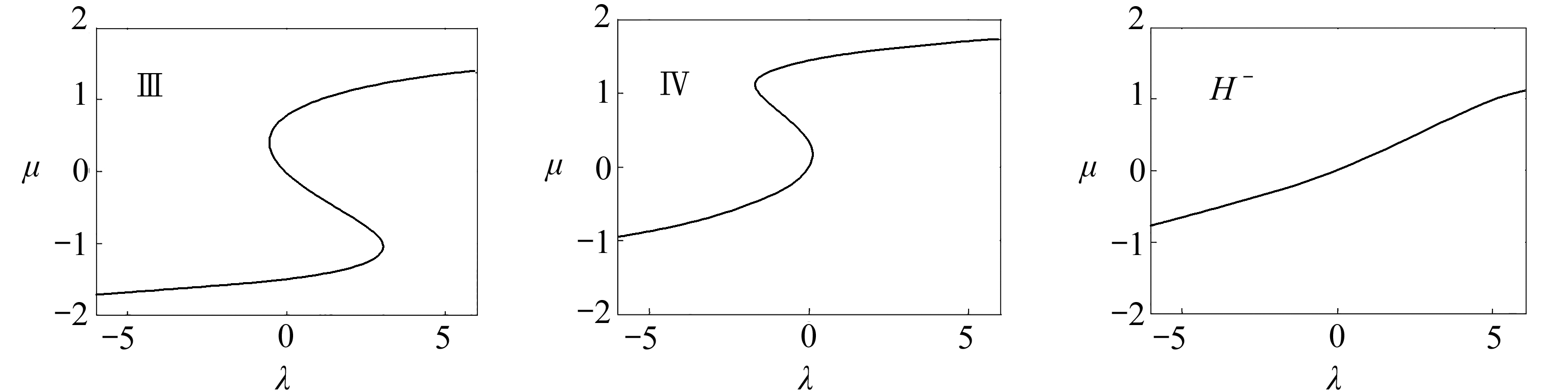Fig. 6 The topological structure of bifurcation curves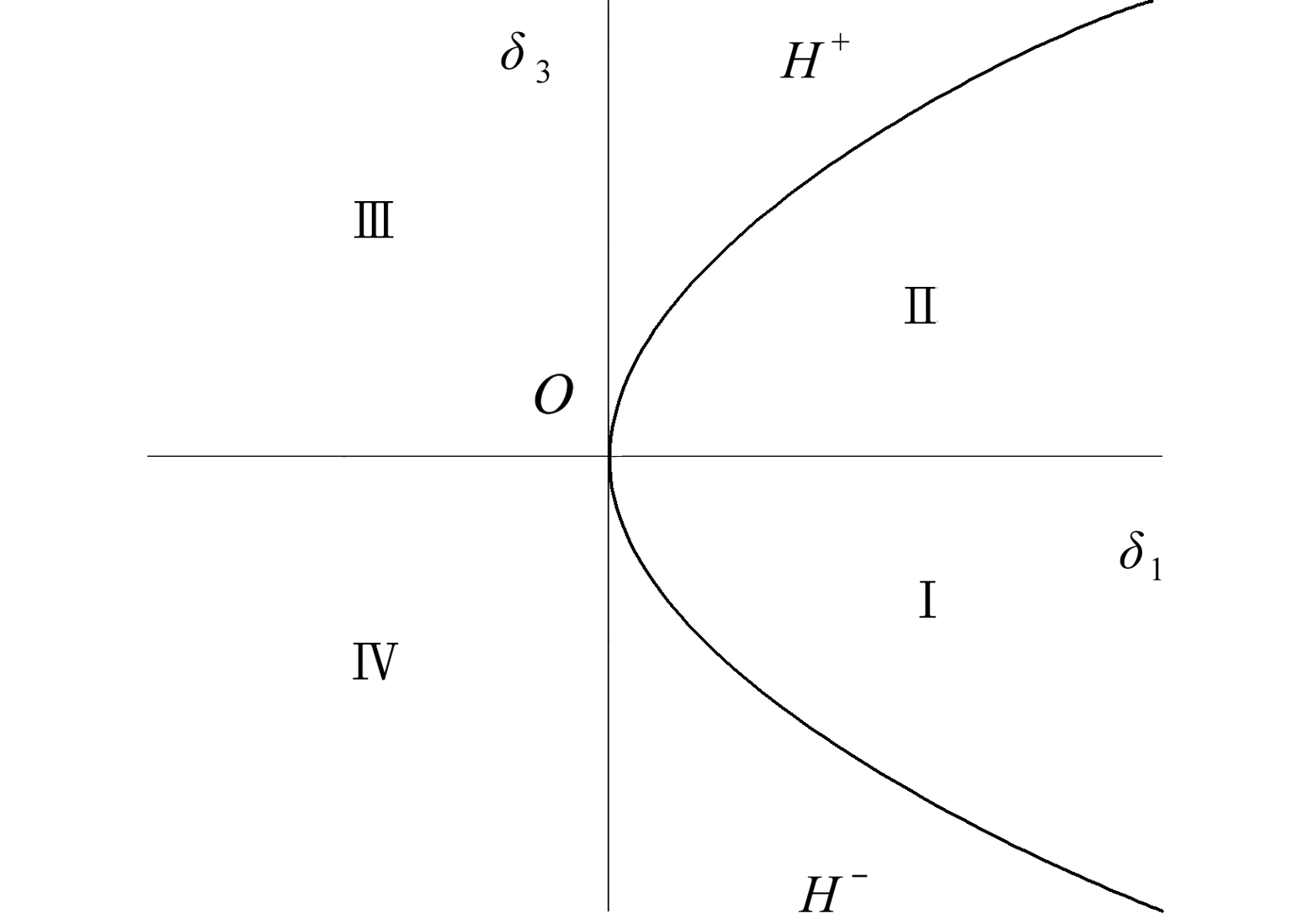Fig. 7 The transition set for δ2=0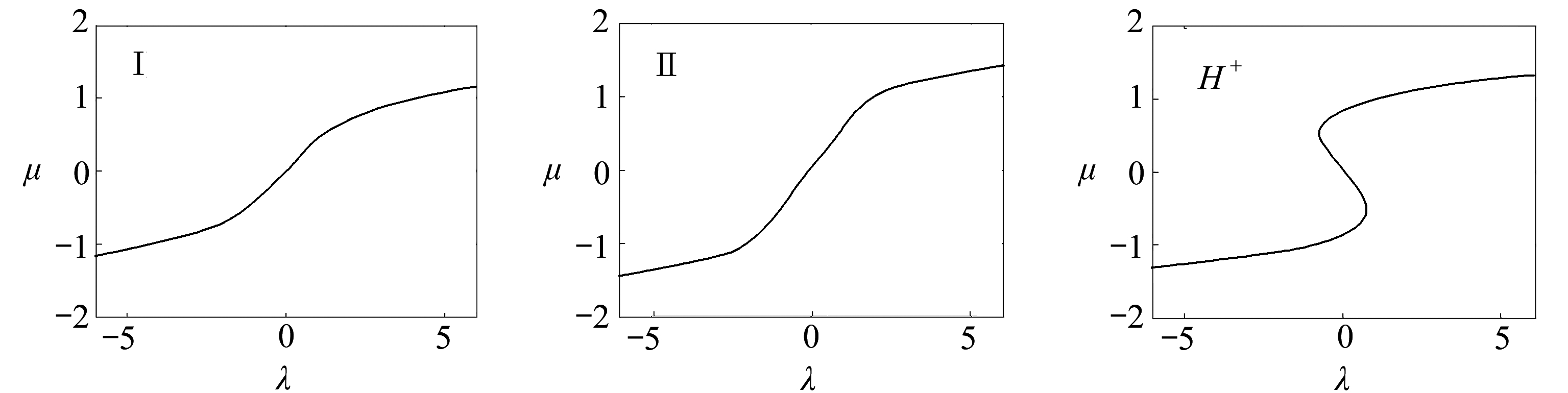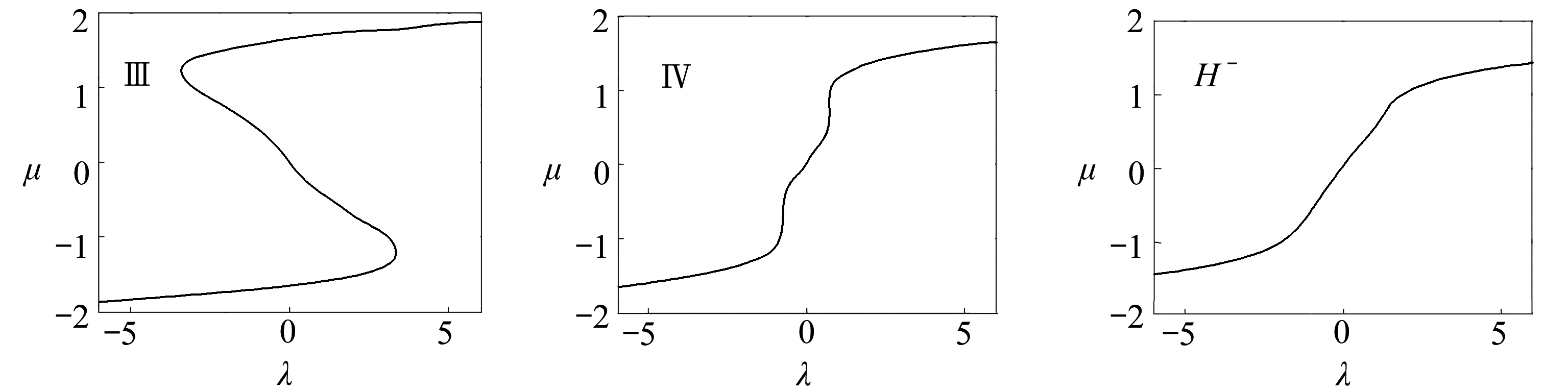Fig. 8 The topological structure of bifurcation curves

δ2=0

δ2=0时，可得系统分岔点集B=∅，滞后点集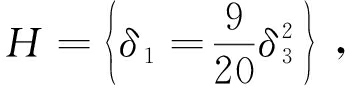δ1=0

δ1=0时，可得系统分岔点集：B=∅；滞后点集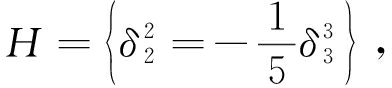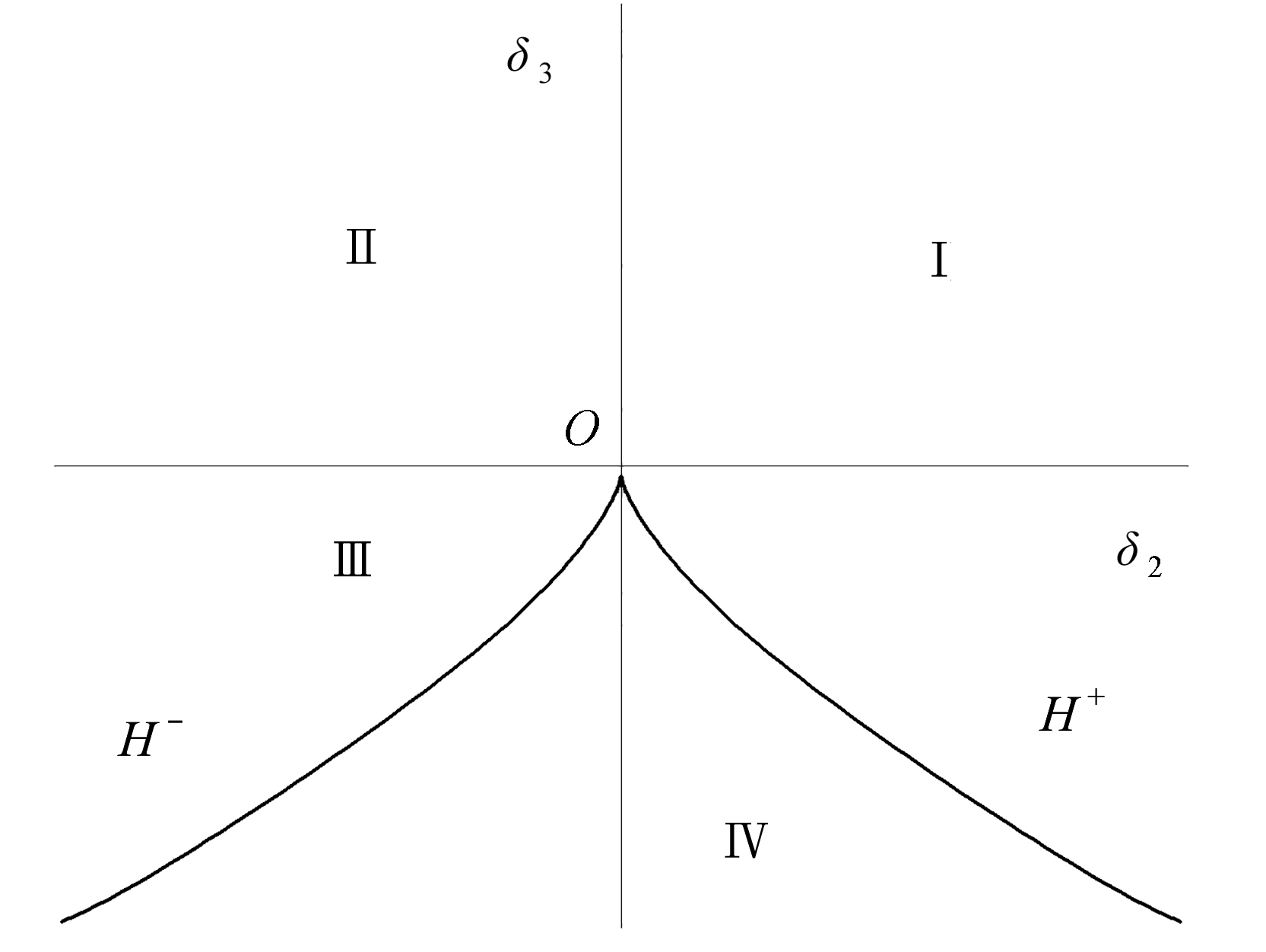Fig. 9 The transition set for δ1=0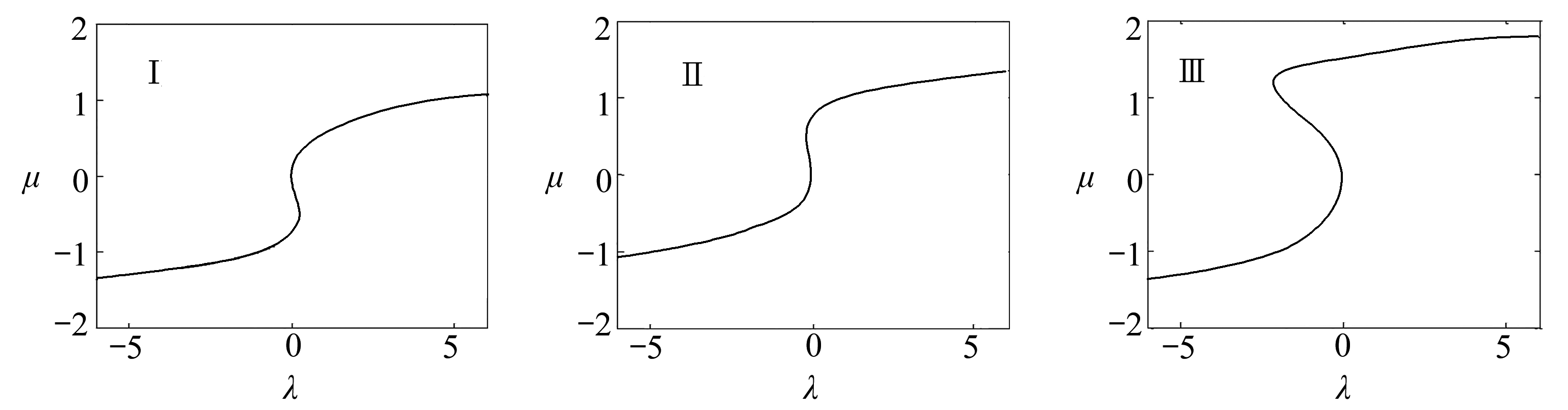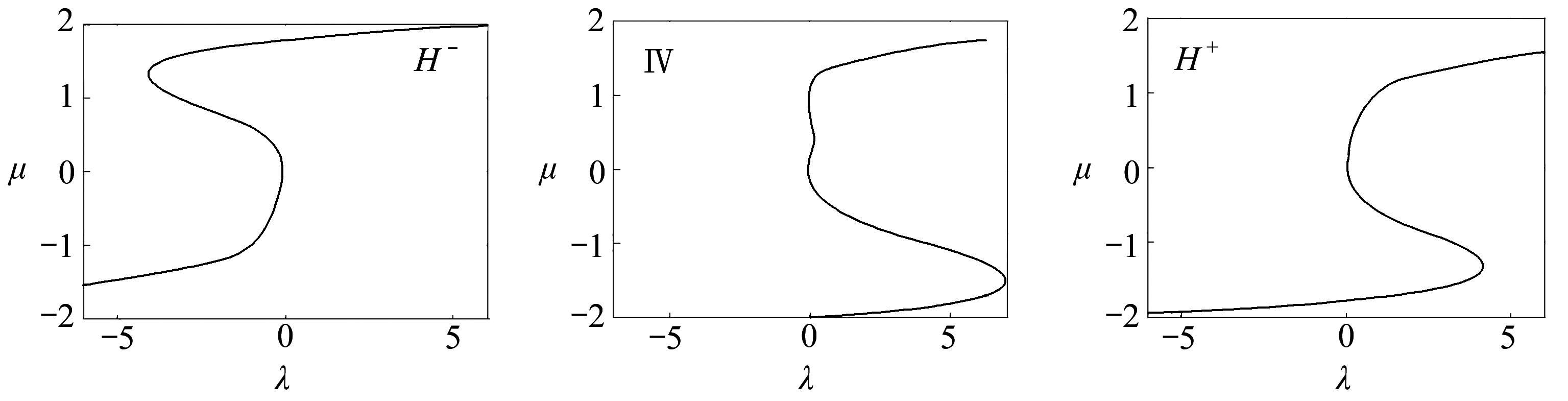Fig. 10 The topological structure of bifurcation curves

## 3 系统动态分岔与混沌运动分析

### 3.1 系统不动点分析及异宿轨道求解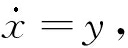则式(1)可写为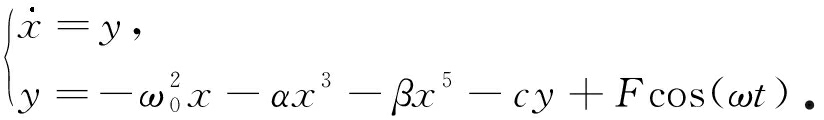(13)(14)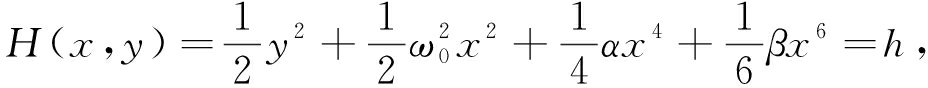(15)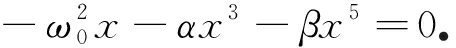(16)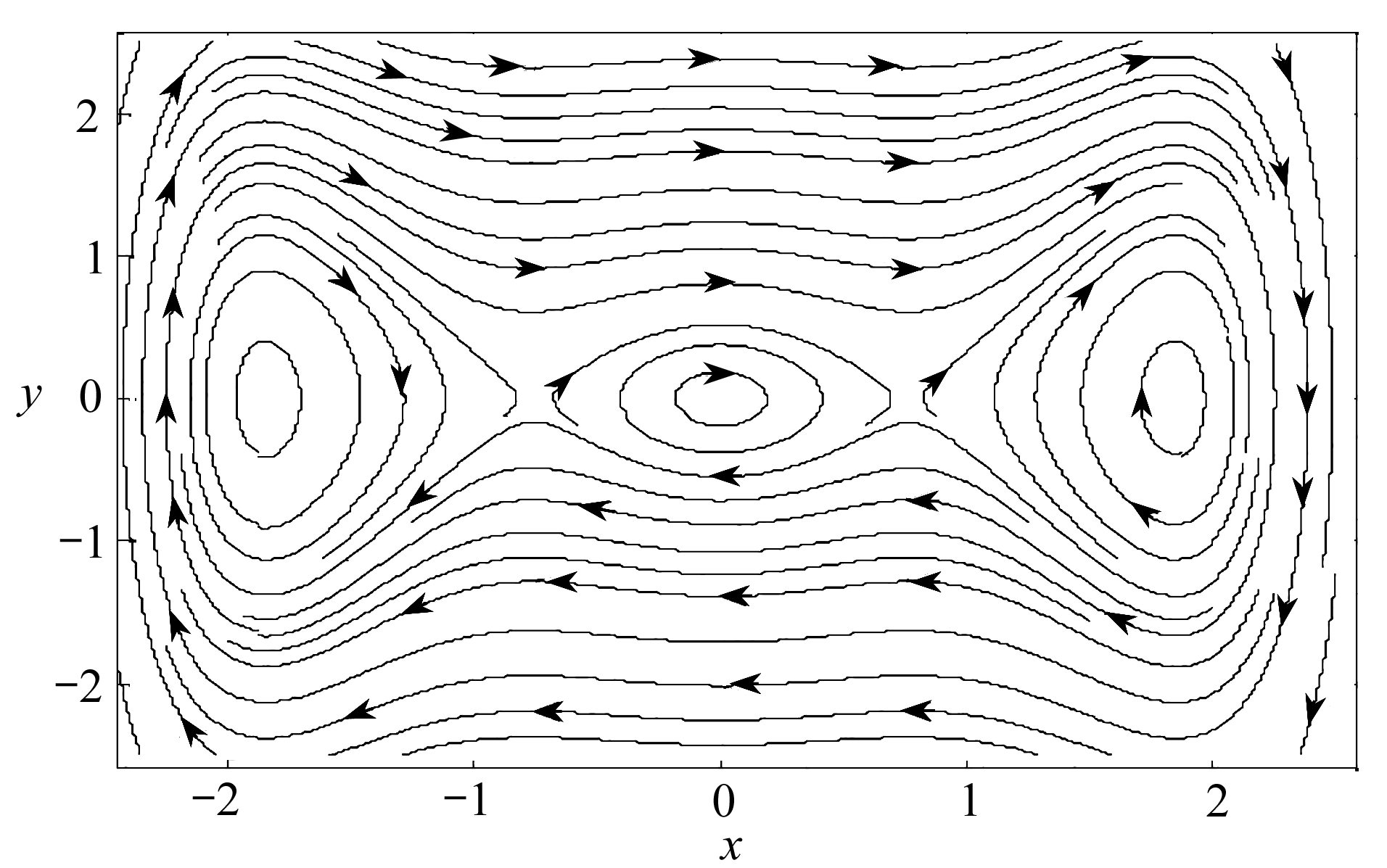Fig. 11 The phase plane of system (13)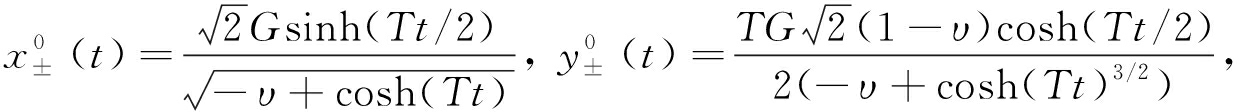(17)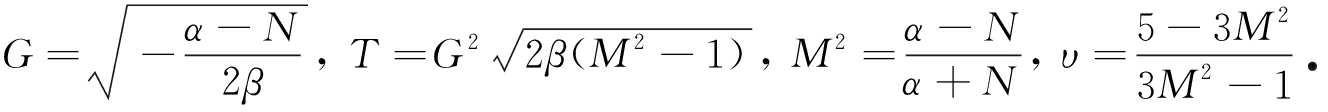### 3.2 混沌阈值求解及预测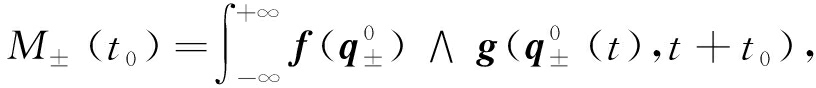(18)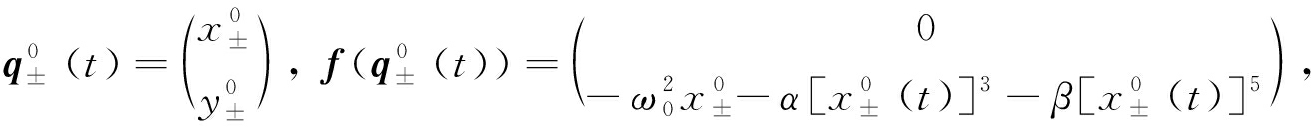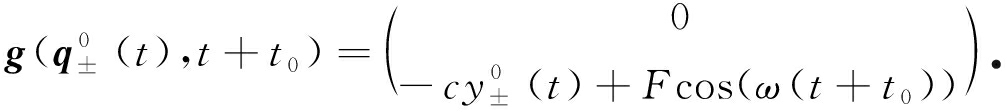M±(t0)=-cI1+FI2cos(ωt0)，

(19)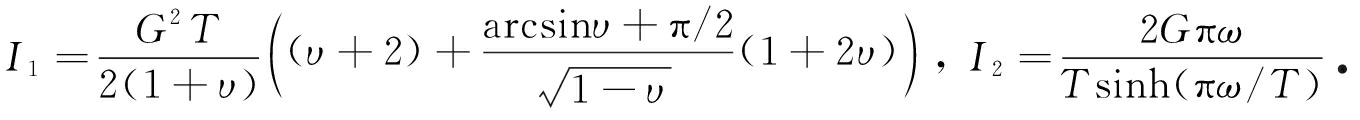M±(t0)=0得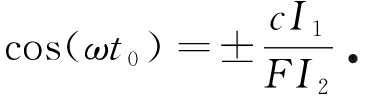(20)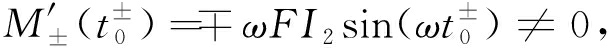(21)

## 4 系统动态分岔与混沌特性仿真分析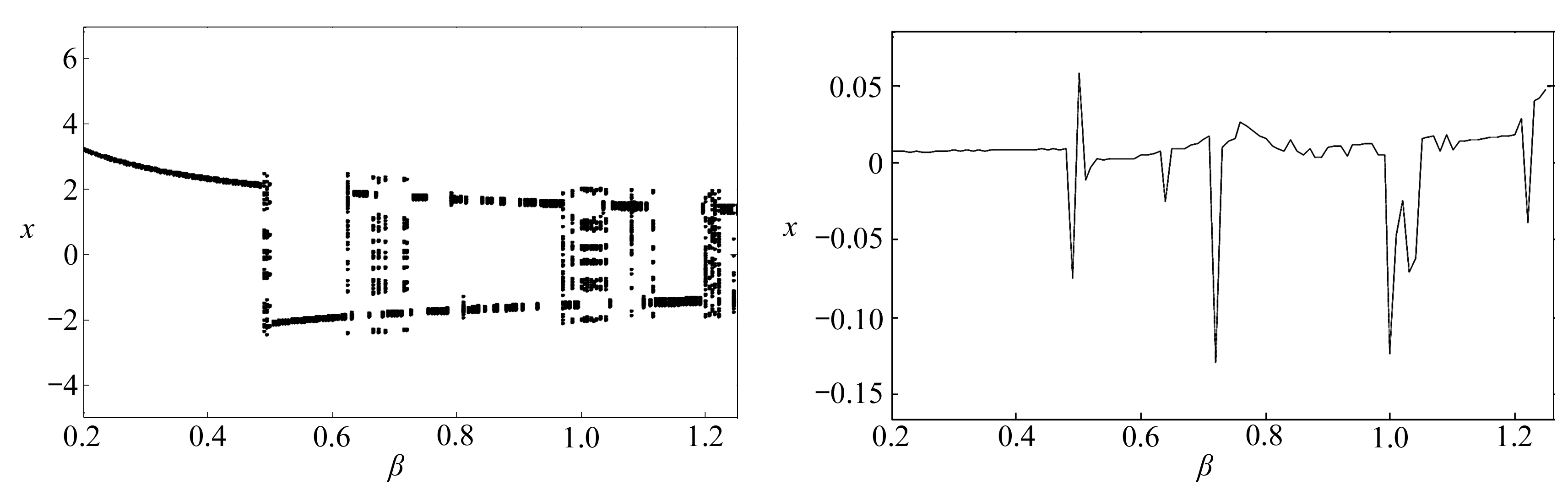Fig. 12 The dynamic bifurcation diagram with quintic Fig. 13 The maximum Lyapunov exponent changing with nonlinear coefficient β of the system quintic nonlinear coefficient β of the system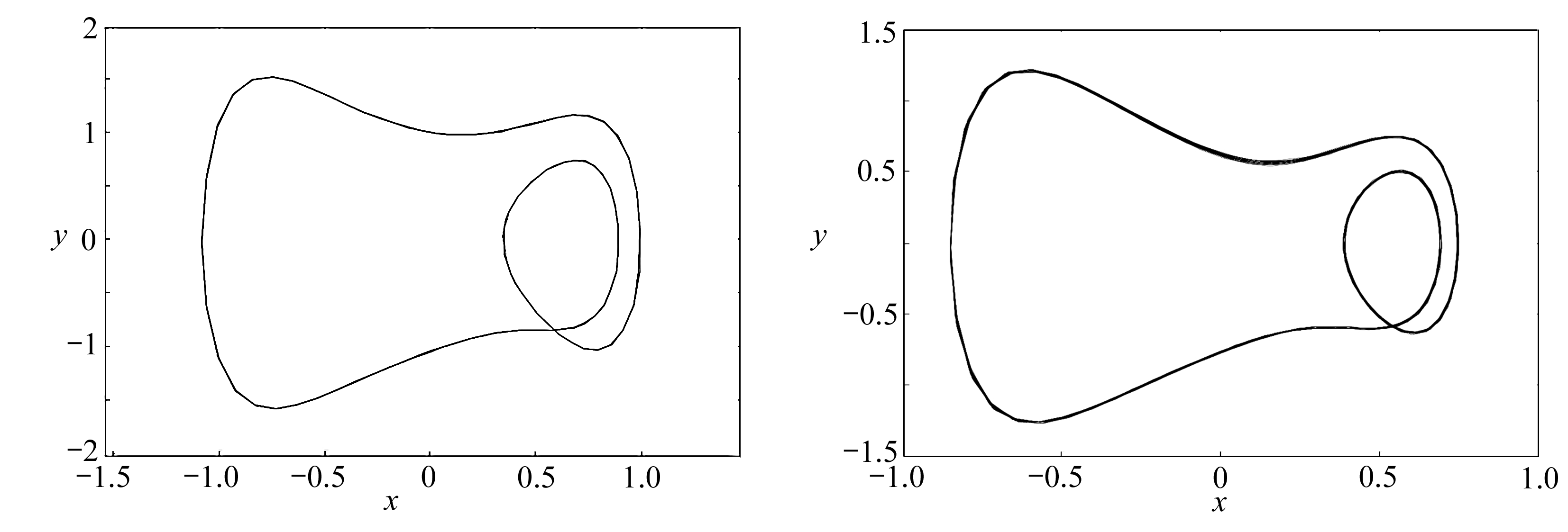(a) β=0.45(b) β=0.88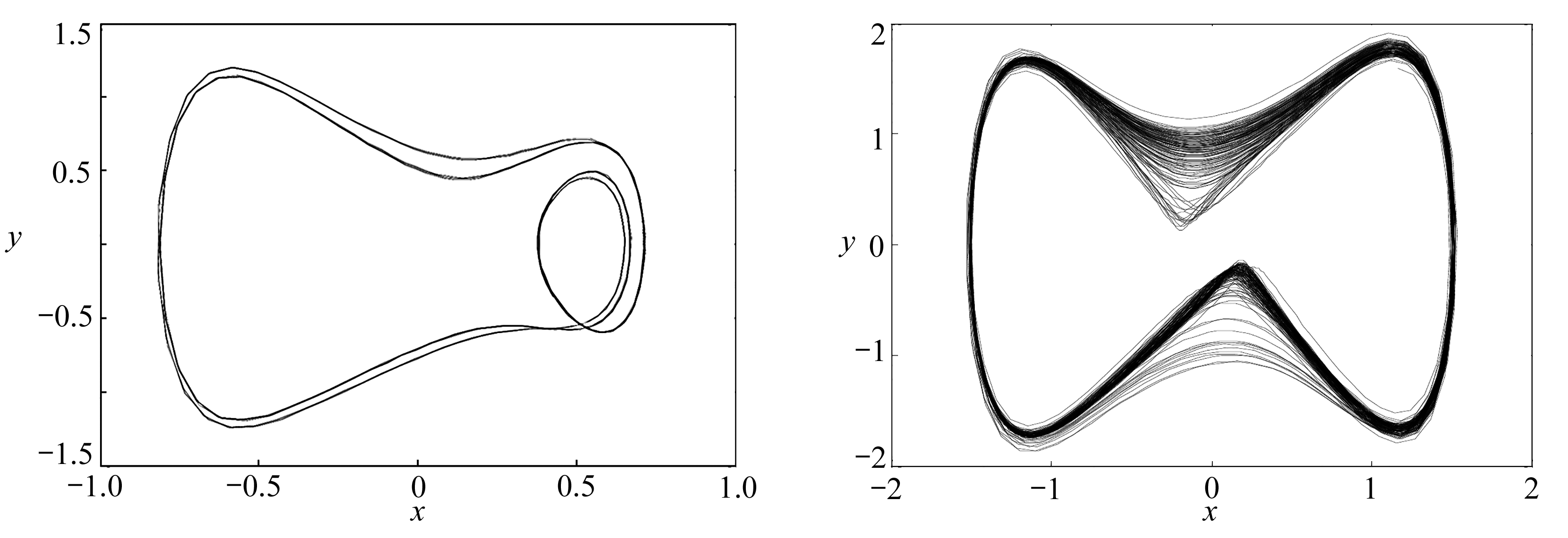(c) β=1.05(d) β=1.22

Fig. 14 The phase diagram changing with quintic nonlinear coefficient β of the system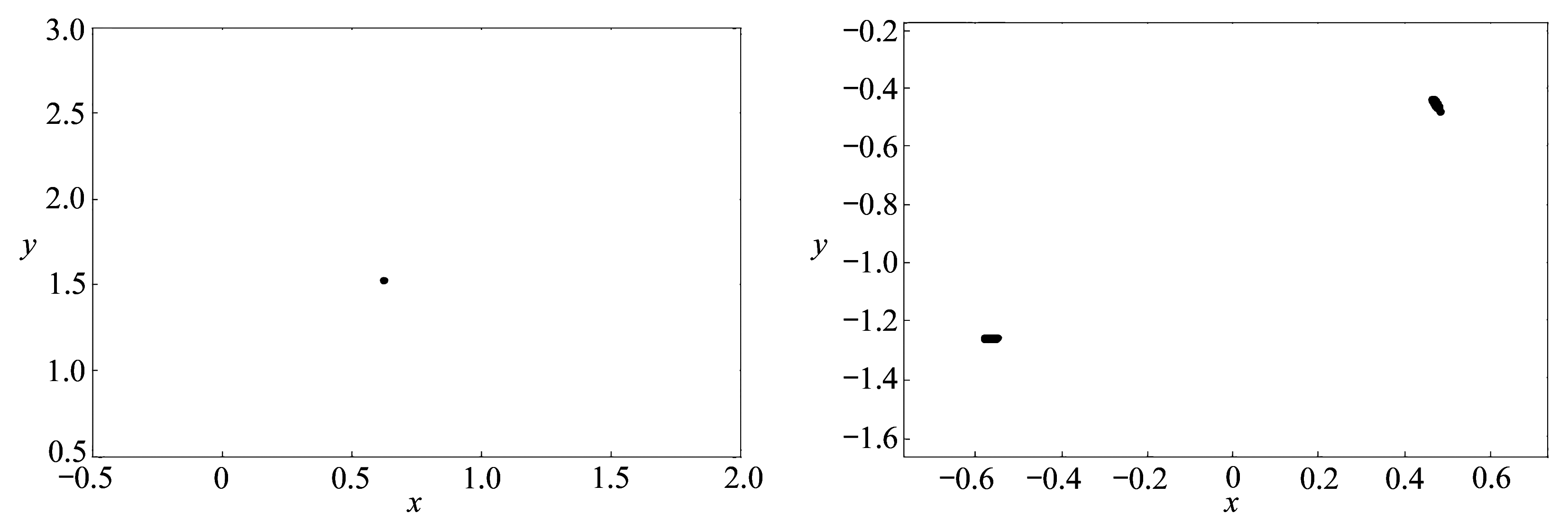(a) β=0.45(b) β=0.88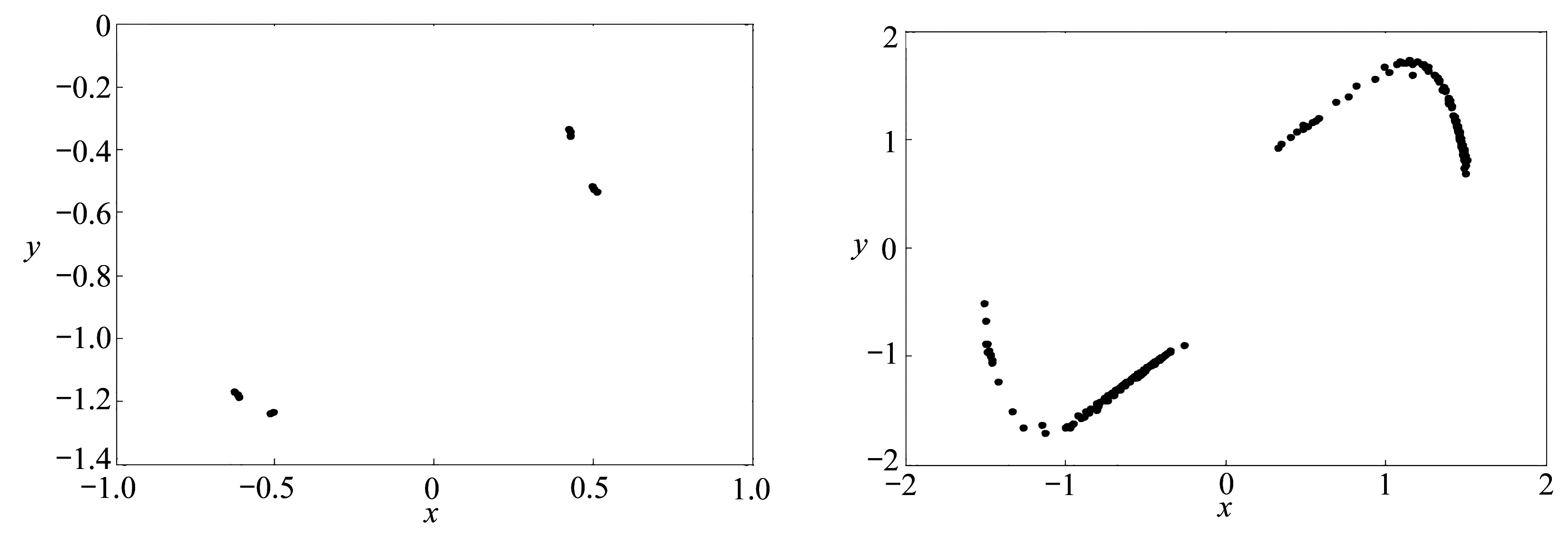(c) β=1.05(d) β=1.22

Fig. 15 The Poincaré sections changing with quintic nonlinear coefficient β of the system

### 4.2 外激力F变化时系统的分岔特性分析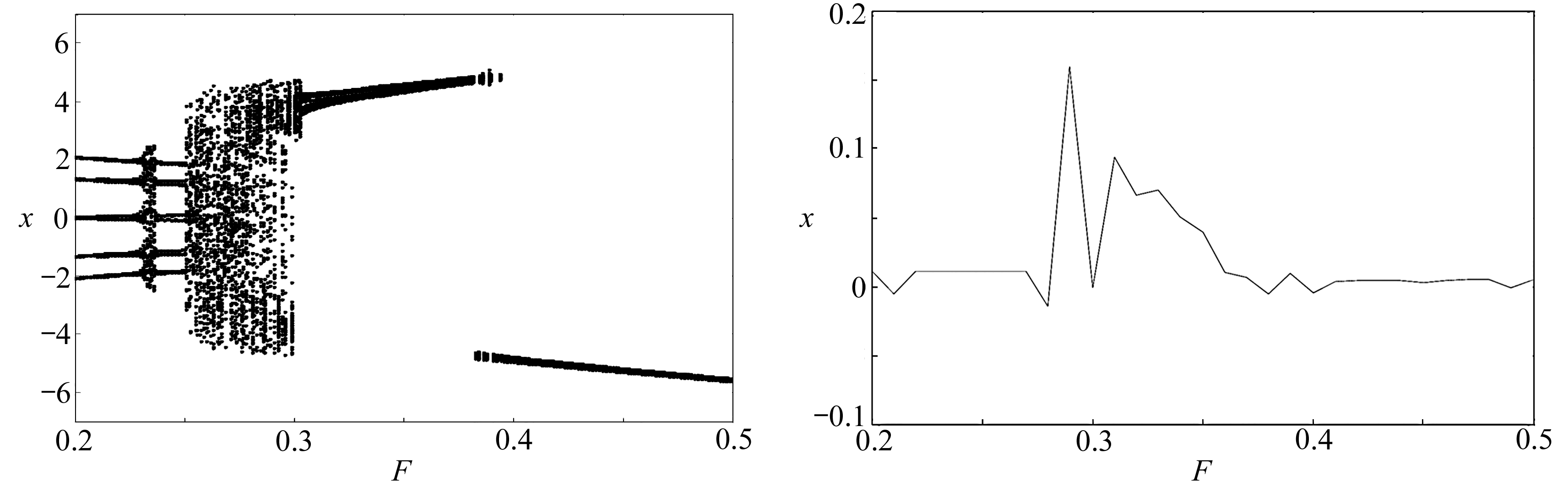Fig. 16 The dynamic bifurcation diagram with Fig. 17 The maximum Lyapunov exponent changing
excitation amplitude F of the system with excitation amplitude F of the system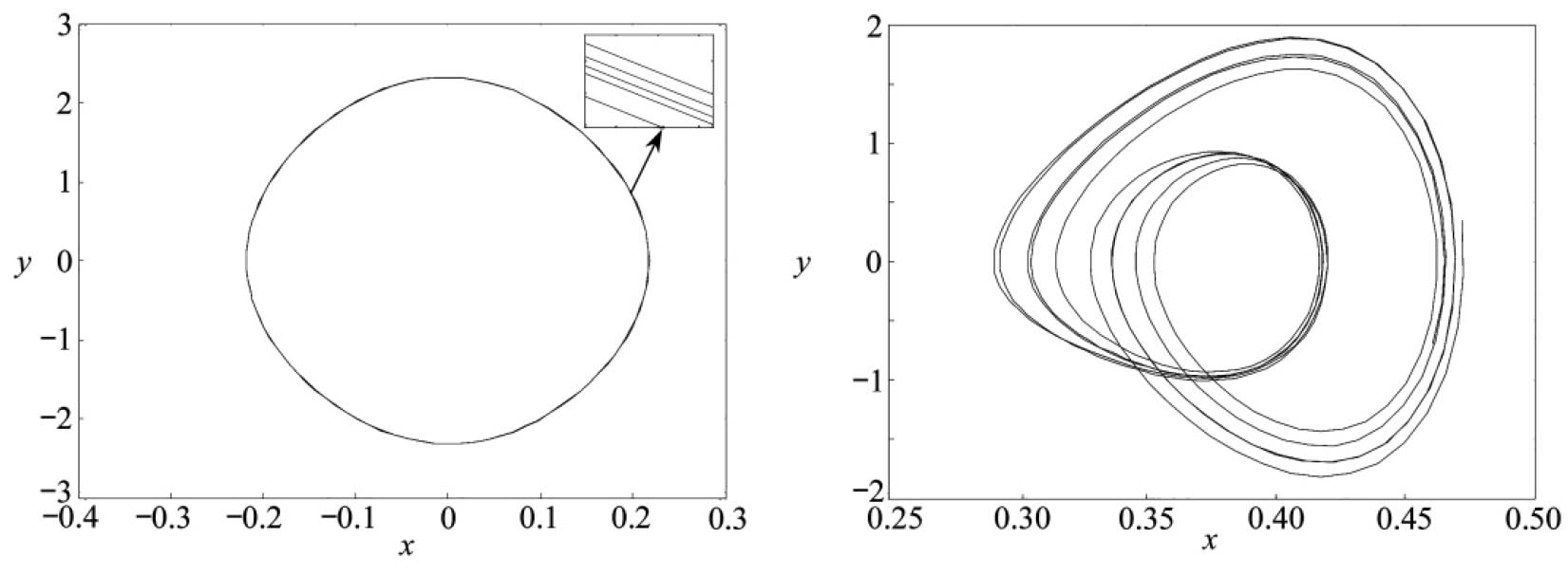(a) F=0.21(b) F=0.25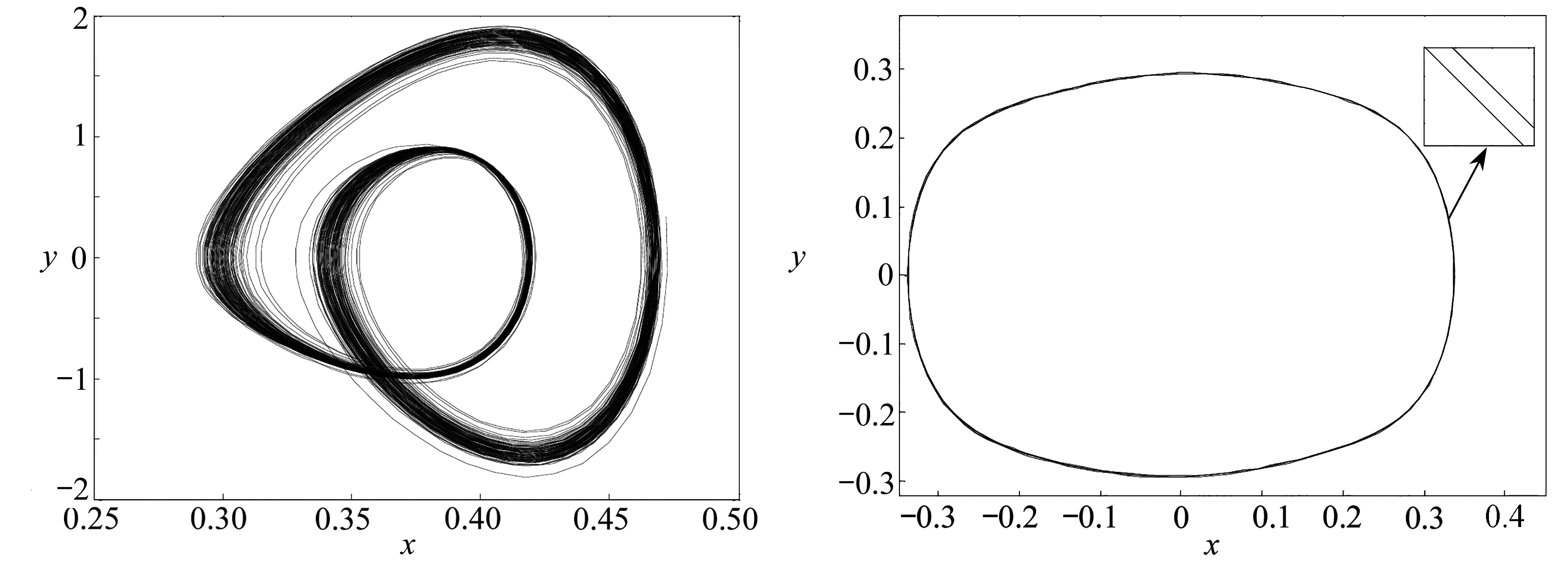(c) F=0.28(d) F=0.40

Fig. 18 The phase diagram changing with excitation amplitude F of the system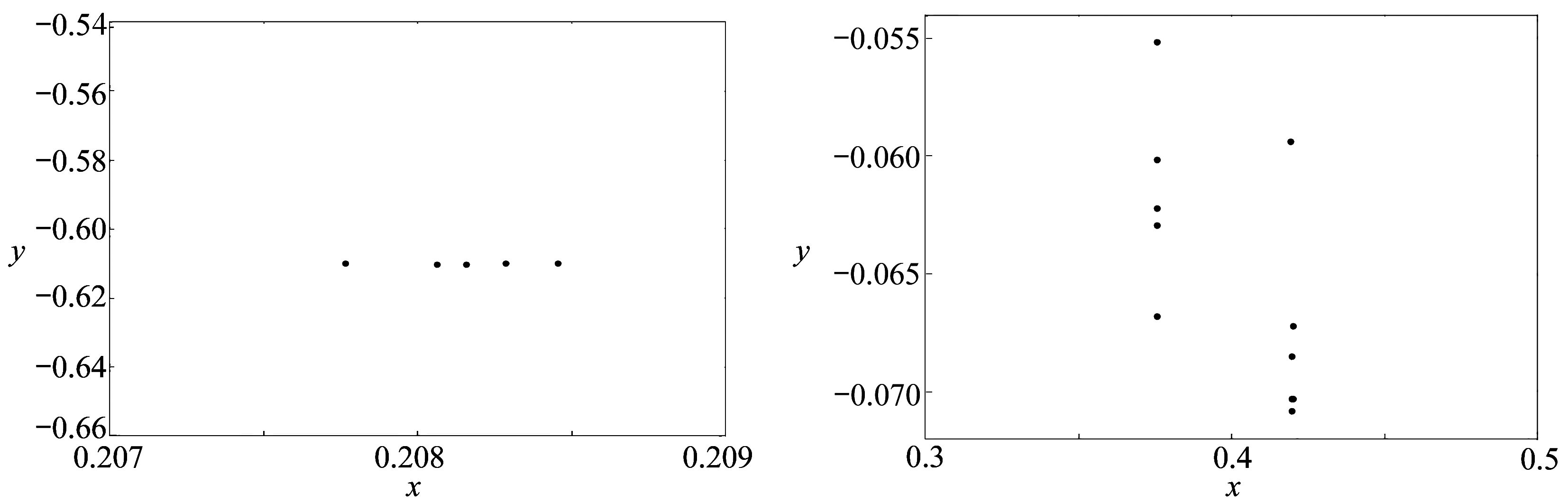(a) F=0.21(b) F=0.25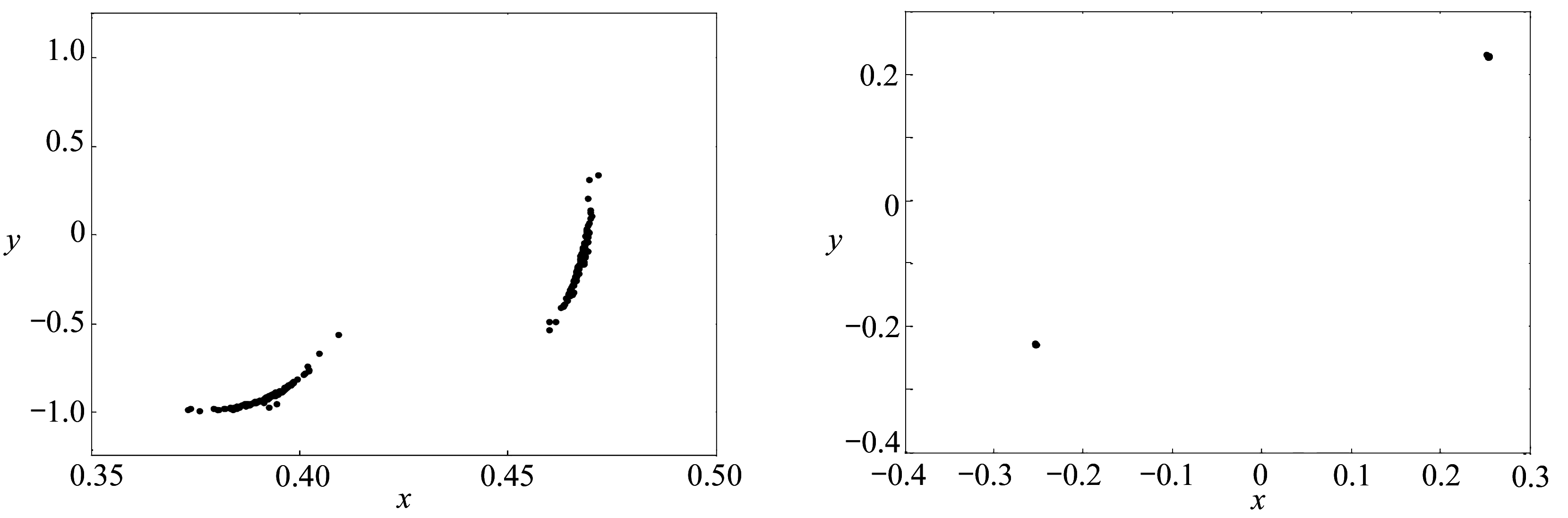(c) F=0.28(d) F=0.40

Fig. 19 The Poincaré sections changing with excitation amplitude F of the system

## 5 结 论

 MOON F C, HOLMES P J. A magnetoelastic strange attractor[J]. Journal of Sound and Vibration, 1979, 65(2): 285-296.

 MOON F C, HOLMES P J. Addendum: a magnetoelastic strange attractor[J]. Journal of Sound and Vibration, 1980, 69(2): 339.

 HOLMES C, HOLMES P. Second order averaging and bifurcations to subharmonics in Duffing’s equation[J]. Journal of Sound and Vibration, 1981, 78(2): 161-174.

 LAZER A C, MCKENNA P J. On the existence of stable periodic solutions of differential equations of Duffing type[J]. Proceedings of the American Mathematical Society, 1990, 110: 125-133.

 王坤, 关新平, 丁喜锋, 等. Duffing振子系统周期解的唯一性与精确周期信号的获取方法[J]. 物理学报, 2010, 59(10): 6859-6863.(WANG Kun, GUAN Xinping, DING Xifeng, et al. Acquisition method of precise periodic signal and uniqueness of periodic solutions of Duffing oscillator system[J]. Acta Physica Sinica, 2010, 59(10): 6859-6863.(in Chinese))

 韩祥临, 林万涛, 许永红, 等. 广义Duffing扰动振子随机共振机理的渐近解[J]. 物理学报, 2014, 63(17): 35-39.(HAN Xianglin, LIN Wantao, XU Yonghong, et al. Asymptotic solution to the generalized Duffing equation for disturbed oscillator in stochastic resonance[J]. Acta Physica Sinica, 2014, 63(17): 35-39.(in Chinese))

 YAGASAKI K. Homoclinic tangles, phase locking, and chaos in a two-frequency perturbation of Duffing’s equation[J]. Journal of Nonlinear Science, 1999, 9(1): 131-148.

 刘延柱, 陈立群. 非线性振动[M]. 北京: 高等教育出版社, 2001.(LIU Yanzhu, CHEN Liqun. Nonlinear Vibrations[M]. Beijing: Higher Education Press, 2001.(in Chinese))

 LORENZ E N. Deterministic nonperiodic flow[J]. Journal of the Atmospheric Sciences, 1963, 20: 130-141.

 NOVAK S, FREHLICH R G. Transition to chaos in the Duffing oscillator[J]. Physical Review, 1982, 26(6): 3660-3663.

 STAGLIANO J J, WERSINGER J M, SLAMINKA E E. Doubling bifurcation of destroyed T2 tori[J]. Physica D: Nonlinear Phenomena, 1996, 92(3/4): 164-177.

 HUANG J, JING Z. Bifurcation and chaos in the three-well Duffing equation with one external forcing[J]. Chaos Solition and Fractels, 2009, 40(3): 1449-1466.

 侯东晓, 赵红旭, 刘彬. 一类含Mathieu-Duffing振子的相对转动系统的分岔和混沌[J]. 物理学报, 2013, 62(23): 232-242.(HOU Dongxiao, ZHAO Hongxu, LIU Bin. Bifurcation and chaos in some relative roration systems with Mathieu-Duffing oscillator[J]. Acta Physica Sinica, 2013, 62(23): 232-242.(in Chinese))

 张莹, 都琳, 岳晓乐, 等. 随机参数作用下参激双势阱Duffing系统的随机动力学行为分析[J]. 应用数学和力学, 2016, 37(11): 1198-1207.(ZHANG Ying, DU Lin, YUE Xiaole, et al. Stochastic nonlinear dynamics analysis of double-well Duffing systems under random parametric excitations[J]. Applied Mathematics and Mechanics, 2016, 37(11): 1198-1207.(in Chinese))

 张艳龙, 王丽, 石建飞. Duffing系统在双参数平面上的分岔演化过程[J]. 应用数学和力学, 2018, 39(3): 324-333.(ZHANG Yanlong, WANG Li, SHI Jianfei. Bifurcation evolution of Duffing systems on 2-parameter planes[J]. Applied Mathematics and Mechanics, 2018, 39(3): 324-333.(in Chinese))

 姚海洋, 王海燕, 张之深, 等. 一种基于广义Duffing振子的水中弱目标检测方法[J]. 物理学报, 2017, 66(12): 227-238.(YAO Haiyang, WANG Haiyan, ZHANG Zhishen, et al. A method of detecting underwater weak target based on generalized Duffing oscillator[J]. Acta Physica Sinica, 2017, 66(12): 227-238.(in Chinese))

 刘莉, 徐伟, 岳晓乐, 等. 一类含非黏滞阻尼的Duffing单边碰撞系统的激变研究[J]. 物理学报, 2013, 62(20): 200501.(LIU Li, XU Wei, YUE Xiaole, et al. Global analysis of crises in a Duffing vibro-impact oscillator with non-viscously damping[J]. Acta Physica Sinica, 2013, 62(20): 200501.(in Chinese))

 唐友福, 刘树林, 雷娜, 等. 基于广义局部频率的Duffing系统频域特征分析[J]. 物理学报, 2012, 61(17): 67-75.(TANG Youfu, LIU Shulin, LEI Na, et al. Feature analysis in frequency domain of Duffing system based on general local frequency[J]. Acta Physica Sinica, 2012, 61(17): 67-75.(in Chinese))

 RONG H W, XU W, FANG T. Principal response of Duffing oscillator to combined deterministic and narrow-band random parametric excitation[J]. Journal of Sound and Vibration, 1998, 210(4): 483-515.

# AnalysisofResonanceandBifurcationCharacteristicsofSomeDuffingSystemsWithQuinticNonlinearRestoringForces

PENG Rongrong

(School of Sciences, Nanchang Institute of Science & Technology, Nanchang 330108, P.R.China)

Abstract: Some Duffing systems with external excitation and quintic nonlinear restoring forces were considered, the amplitude-frequency response equation for the system was obtained with the multi-scale method, and the amplitude-frequency characteristic curves and their changing rules under different parameter changes were given. At the same time, the singularity theory was applied to get the transition sets and the corresponding topological structures of the system in 3 cases. Second, the fixed point of the system was determined, and the Hamiltonian function was used to get the heteroclinic orbit of the system, so the threshold of chaos in the Smale horseshoe sense was obtained with the Melnikov method. Then, the dynamic bifurcation and chaotic behavior of the system under external excitation and quintic nonlinear coefficients were given through numerical simulation. It is found that there are nonlinear phenomena such as periodic motion, period doubling motion, quasi periodic motion and chaos. The correctness of the theory was verified with nonlinear methods such as the Lyapunov exponent, the phase diagram and the Poincaré sections. The work provides a theoretical reference for further understanding of the nonlinear characteristics of Duffing systems and their evolution laws.

Key words: Duffing system; quintic nonlinearity; bifurcation; chaos

ⓒ 应用数学和力学编委会，ISSN 1000-0887

*收稿日期： 2018-09-04； 修订日期：2018-11-28

DOI: 10.21656/1000-0887.390234